Courses

# Test: Packing Crystals

## 22 Questions MCQ Test Chemistry Class 12 | Test: Packing Crystals

Description
This mock test of Test: Packing Crystals for Class 12 helps you for every Class 12 entrance exam. This contains 22 Multiple Choice Questions for Class 12 Test: Packing Crystals (mcq) to study with solutions a complete question bank. The solved questions answers in this Test: Packing Crystals quiz give you a good mix of easy questions and tough questions. Class 12 students definitely take this Test: Packing Crystals exercise for a better result in the exam. You can find other Test: Packing Crystals extra questions, long questions & short questions for Class 12 on EduRev as well by searching above.
QUESTION: 1

### Only One Option Correct Type This section contains 11 multiple choice questions. Each question has four choices (a), (b), (c) and (d), out of which ONLY ONE is correct. Q. CsCI crystallises in a cube that has CP at each corner and Cs+ at the centre of the unit cell. If  rCs+  = 169 pm and rcl- =181 pm, then edge length of the cube is

Solution:

(d) CsCI has bcc structure.
Closest dista nce between Cs+ and Cl- is the sum of radii of Cs+ and CI- = 169 + 181 = 350 pm

Cubic diagonal = √3 a

Closest distance is one-half of the cubic diagonal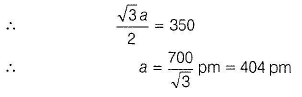QUESTION: 2

### A mineral having the formula AB2 crystallises in the cubic closest - packed lattice with the A-atoms occupying the lattice points. Fraction of the tetrahedral sites occupied by 6-atoms is

Solution:

(d) ABcrystallises as cubic close packed (ccp).

Thus, A2+ is surrounded by eight B- and B- is surrounded by four A2+ .

Thus, coordinatio n number of A2+ = B

coordination number of B- = 4

Number of atoms in tetrahedral in small cube of unit cell of atom B = 4

Thus, fraction occupied = 4/4 = 1 (100%)

QUESTION: 3

### Silver metal crystallises in a cubic closest packed arrangement with edge length 407 pm. Thus, radius of the silver atom is

Solution:

(c)

AB = a, AC = a

BC2 = AB2 + AC2 =2a2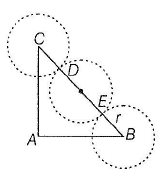then BC = CD + DE + EB = r + 2r + r = 4r

∴ 16r2 = 2a2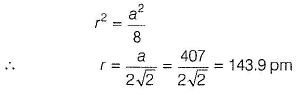QUESTION: 4

What is the formula of a magnetic oxide of cobalt used in recording tapes that crystallises with cobalt atoms occupying one-eighth of the tetrahedral holes and one-half of the octahedral holes in a closest packed array of oxide ions?

Solution:

(c)

Tetrahedral holes = 8, Octahedral holes = 4 Cobalt atoms = 8/8 =1

Oxide ions = 4/2 =2

Thus, formula is CoO2

QUESTION: 5

The total number of tetrahedral voids in the face-centred unit cell is

Solution:

(b) Unit cell of fee is divided into eight small cubes. Each cube has four atoms at alternate positions. When joined to each other, they make a regular tetrahedron.
Thus, number of tetrahedral void in small cube = 1 and in eight cubes, voids = 8.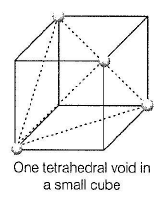Note  If number of atoms in a cube = N, then, number of octahedral voids = N and number of tetrahedral voids = 2N

QUESTION: 6

Packing efficiency (%) of different types of unit cells is given. Select the correct packing efficiency.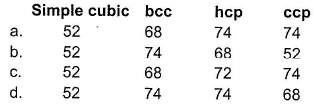Solution:

(a) Edge length = a, Volume of one face = a3 Volume of six faces of a cube = 6a3 = V Radius = r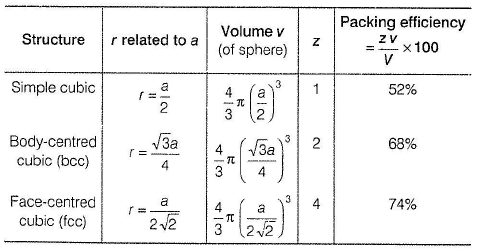fee can be hexagonal closest packed (hep)

or cubic closest packed (ccp)

z = number of atoms in unit cell.

QUESTION: 7

Coordination numbers in a square close packed structure and hexagonal close packed structure respectively, are

Solution:

(c) Close packing in two-dimensions can be Type I A A A ...... ty p e The second row may be ^ placed in contact with the first one such that the spheres of A the second row are exactly . above those of the first row.
The spheres of the two rows A are aligned horizontally as well as vertically. In this arrangement, each sphere is in contact with four of its neighbours.
Thus, its coordination number = 4 and is called square close packing in two-dimensions.
Atom A (•) is in contact with four others.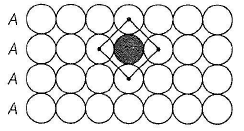Type II ABAB... type The second row may be placed above the first in a staggered manner such that its spheres fit in the depressions of the first row.
First row arrangement: A type Second row arrangement: 8 type Third row arrangement: A type and so on There is less free space and packing is more efficient than the square close packing. Each sphere is in contact with six of its neighbours and coordination number = 6 in two-dimensions. It is called two dimensional hexagonal close-packing. Voids formed in this case are triangular in shape. If the apex of the triangles downward in the first row, it is upward in the second row.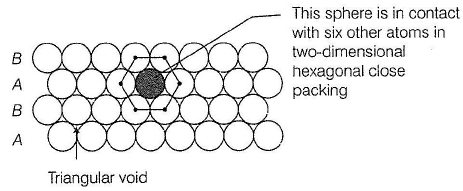QUESTION: 8

The length of the unit cell edge of a bcc lattice metal is 352 pm. Thus, volume of atoms in one mole of the metal is

Solution:

(a)

In a bcc structure, the atoms tou ch each other along the body diagonal.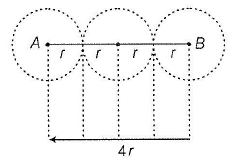Radius of the atom = r

AB2 = 8C2 + AC2
BC2 = BD2 + CD2 = a2 + a2 = 2a2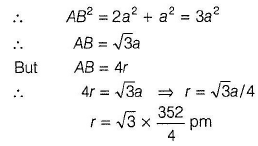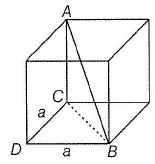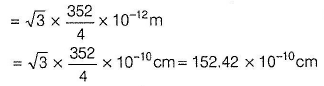Volume of one atom =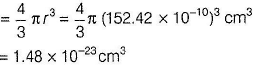Volume of N0 atoms in one mole of the metal

= 6.02 x 1023 x 1.48 x 10-23 cm3 = 8.93 cm3

QUESTION: 9

A compound Mp Xq has cubic close packing arrangement of X. Its unit cell structure is shown below. The empirical formula of the compound is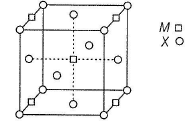Solution:

(b)

Structure is cubic close packing.
Number of atoms of M =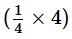(from edge centre) +1 (from body centre) =2

Number of atoms of X =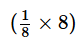(from corners of the cube) +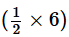(from face centre)  =4

Thus, M : X = 2 : 4 = 1 : 2 Hence, the empirical formula of the compound is MX2.

QUESTION: 10

Radii of A+ and that of X- and Y- have been given as

A+= 1.00 pm

X- = 1.00 pm

Y- = 2.00 pm

Thus, ratio of volumes of A X and A Y unit cells is

Solution:

(a) Ratio of r+ /r_ will decid e the type of unit cell

For AX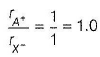hence, AX has bcc structure.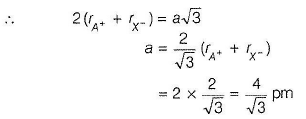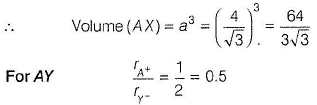Thus, octahedral or square planar structure.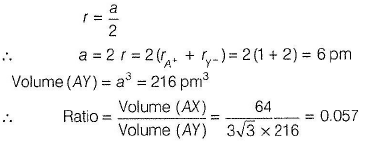QUESTION: 11

In the structure of NaCI given below, ratio rNa+/rcl is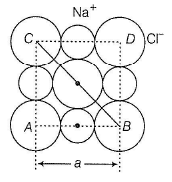Solution: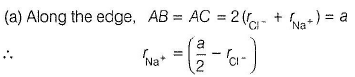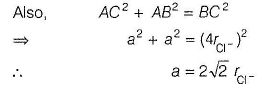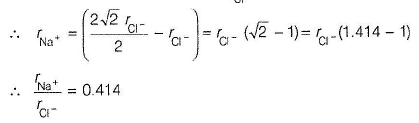QUESTION: 12

Matching List Type
Choices for the correct combination of elements from Column I and Column II are given as options (a), (b), (c) and (d), out of which one is correct.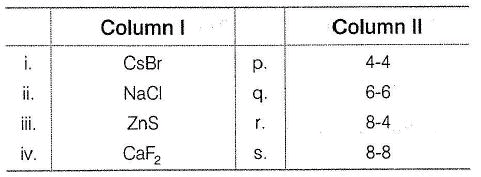Solution:

(i)

Body-centred cubic Every cation (Cs+) is coordinated to eight anions (Br- ) and every anion (Br- ) is coordinated to eight cations (Cs+).
Thus, (i) -> (s)

(ii) Face-centred cubic Every cation (Na+) is coordinated to six anions (Cl- ) and every anion (Cl- ) is coordinated to six cations (Na+).
Thus, (ii) -> (q)

(iii) Face-centred cubic (tetrahedral void) Every cation (Zn2+) is coordinated to four anions (S2-) and vice-versa.
Thus, (iii) —> (p)

(iv) Face-centred cubic (antifluorite) EveryCa2+ is coordinated to eight fluoride and every F- is coordinated to fourCa2+ (to balance charge).

4Ca2+ + 8F-
Thus, (iv) —> (r)

*Multiple options can be correct
QUESTION: 13

One or More than One Options Correct Type
This section contains 5 multiple type questions. Each question has 4 choices (a), (b), (c) and (d), out of which ONE or MORE THAN ONE are correct.

Q.

In hexagonal close packing,

Solution:

(a,b,c)

(a) r = radius, a = edge length

then for hcp, r  =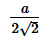Volume of one spherical atom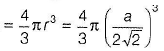Number of atoms in one unit cell = 4

∴ Volume of four atoms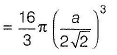Volume of cube = a3

∴ Packing efficiency =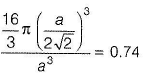Thus, correct.

(b) In hep coordination number = 12, six at the diagonal and six at the edge length thus, correct.

(c) A tetrahedral void is formed when a sphere of a second layer is above the void of the first layer or vice-versa thus, correct.

*Multiple options can be correct
QUESTION: 14

Which of the following are correct about the voids formed in three-dimensional hexagonal close-packed structure?

Solution:

(a, b)

(a) A tetrahedral void is formed when a sphere of a second layer is placed above triangular void in the first layer thus, correct.

(b) All the triangular voids are not covered by this arrangement thus, correct.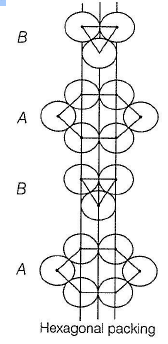*Multiple options can be correct
QUESTION: 15

in which of the following arrangements octahedral voids are formed?

Solution:

(a, d)

Octahedral voids are formed in

(i) hexagonal close packing

(ii) face-centred cubic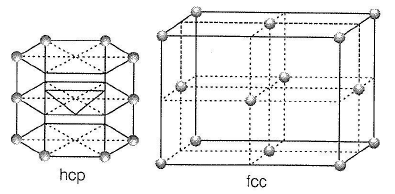QUESTION: 16

Comprehension Type
This section contains a passage describing theory, experiments, data, etc. Two questions related to the paragraph have been given. Each question has only one correct answer out of the given 4 options (a), (b), (c) and (d).

Potassium crystallises in a bcc lattice as shown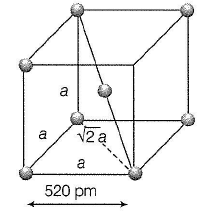Q.

Distance between the two nearest neighbours and between next nearest neighbour respectively, is

Solution:

(a)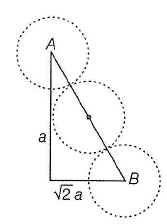Nearest neighbours are along the diagonal at the half distance apart, Diagonal AB = √3a

∴ Distance of the nearest neighbour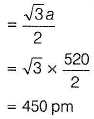Next nearest neighbours are along the cell edge = 520 pm

K-atom at the centre is surrounded by eight K-atoms as nearest neighbours.

In bcc, coordination number is six as next nearest neighbours

QUESTION: 17

Number of neighbours and next - nearest neighbours of K respectively, are

Solution:

(c)Nearest neighbours are along the diagonal at the half distance apart, Diagonal AB = √3a

∴ Distance of the nearest neighbourNext nearest neighbours are along the cell edge = 520 pm

K-atom at the centre is surrounded by eight K-atoms as nearest neighbours.

In bcc, coordination number is six as next nearest neighbours

QUESTION: 18

Metallic gold crystallises in a fee lattice. The length (a) of the cubic unit cell is 407 pm. Atomic mass of gold is 197 amu.

Q.

Closest distance between gold atoms is

Solution:

(a)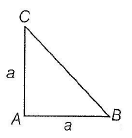Closest distance between two gold atoms is half of the diagonal.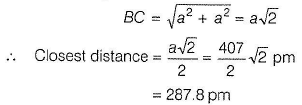Note There are total of 12 nearest neighbours (six along diagonal and six along the edges],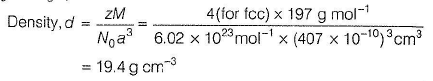QUESTION: 19

Density of gold is

Solution:

(b)Closest distance between two gold atoms is half of the diagonal.Note There are total of 12 nearest neighbours (six along diagonal and six along the edges],*Answer can only contain numeric values
QUESTION: 20

One Integer Value Correct Type
This section contains 3 questions, when worked out will result in an integer value from 0 to 9 (both inclusive).

Atoms of an element B form hep lattice and those o f the element A occupy 2/3rd of tetrahedral voids. How many num ber of atoms of A and B (in total) are present in the hep lattice?

Solution:

Number of atoms in tetrahedral in small cube of unit cell of B = 4
Thus, tetrahedral voids in unit cell of B = 8.

Number of atoms of A = 2/3 of tetrahedral voids of B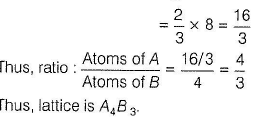Total number of atoms in lattice = 4 + 3 = 7

*Answer can only contain numeric values
QUESTION: 21

How many octahedral voids are formed in one unit cell of a hep or ccp lattice?

Solution:

In fee or hep lattice, body centre is surrounded by six atoms on face centres. If these face centres are joined, one octahedron is formed.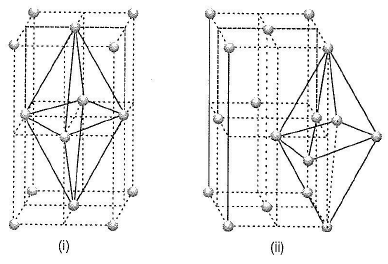Thus, number of octahedral void in the body centre of the cube = 1 There is also one octahedral void at the centre of each of the 12 edges. It is surrounded by six atoms, three belonging to the same unit cell and three belonging to the two adjacent unit cells.

Each edge of the cube is shared between four adjacent unit cells,

thus, number of octahedral voids =12/4 =3

Thus, total octahedral voids =1+ 3 = 4

*Answer can only contain numeric values
QUESTION: 22

Titanium metal has a density of 4.54 g cm-3 and an edge length o f 412.6 pm. How many atoms are there in the unit cell? (Ti = 48)

Solution:

Density (d) =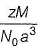where, M = Atomic mass = 48 g mol-1

N0 = Avogadro's number = 6.02 x 1023 m ol-1 !

z = Number of atoms in the unit cell a3 = Volume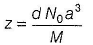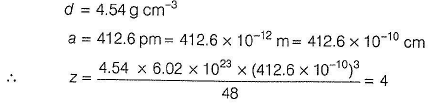Note z = 4 indicates thatTi has fee structure.